# Extended Euclidean Algorithm Example

The Euclidean algorithm is an effective algorithm for finding the greatest common divisor of two integers. It is named after the Greek mathematician Euclid, who invented in VII century.

In the most simple case, Euclidean algorithm is applied to a pair of positive integers and generates a new pair consisting of a smaller number, and the modulo between the larger and the smaller number. The process is repeated until the found number will be the greatest common divisor of the original pair.

The extended Euclidean algorithm also finds such coefficients x and y that: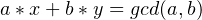Algorithm

To calculate these coefficients we should derive formulas that change them during the transition from pair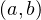to pair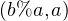(% means the modulo operation)

So, we have a solution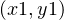for the new pair: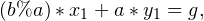And now we want to find a solution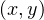for our pair: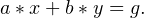To do this we should transform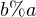: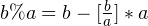Substitute this in the expression below and get: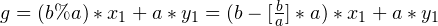Transform: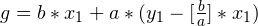So we get the needed expression: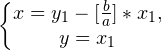C# implementation: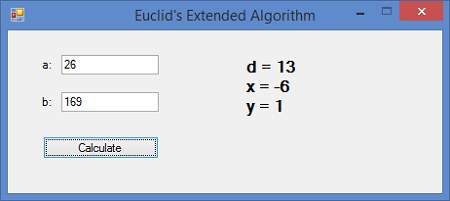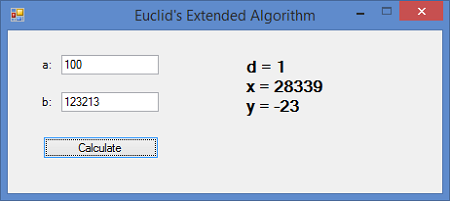Too big values: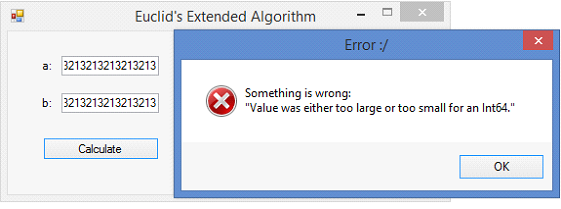Code listing:

EuclidExtended.cs:

using System;

namespace EuclidExtended
{
public class EuclidExtended
{
private long a, b;

public EuclidExtended(long a, long b)
{
this.a = a;
this.b = b;
}

public EuclidExtendedSolution solve()
{
long x0 = 1, xn = 1, y0 = 0, yn = 0, x1 = 0, y1 = 1, f, r = a % b;

while (r > 0)
{
f = a / b;
xn = x0 - f * x1;
yn = y0 - f * y1;

x0 = x1;
y0 = y1;
x1 = xn;
y1 = yn;
a = b;
b = r;
r = a % b;
}

return new EuclidExtendedSolution(xn, yn, b);
}

}
}


Code listing:

EuclidExtended.cs:

using System;

namespace EuclidExtended
{
public class EuclidExtended
{
private long a, b;

public EuclidExtended(long a, long b)
{
this.a = a;
this.b = b;
}

public EuclidExtendedSolution solve()
{
long x0 = 1, xn = 1, y0 = 0, yn = 0, x1 = 0, y1 = 1, f, r = a % b;

while (r > 0)
{
f = a / b;
xn = x0 - f * x1;
yn = y0 - f * y1;

x0 = x1;
y0 = y1;
x1 = xn;
y1 = yn;
a = b;
b = r;
r = a % b;
}

return new EuclidExtendedSolution(xn, yn, b);
}

}
}


EuclidExtendedSolution.cs:

using System;

namespace EuclidExtended
{
public class EuclidExtendedSolution
{
private long x, y, d;

public long X
{
get
{
return this.x;
}
}

public long Y
{
get
{
return this.y;
}
}

public long D
{
get
{
return this.d;
}
}

public EuclidExtendedSolution(long x, long y, long d)
{
this.x = x;
this.y = y;
this.d = d;
}
}
}



Form1.Designer.cs:

namespace EuclidExtended
{
partial class Form1
{
///

/// <summary>
/// Required designer variable.
/// </summary>

private System.ComponentModel.IContainer components = null;

///

/// <summary>
/// Clean up any resources being used.
/// </summary>

/// <param name="disposing">true if managed resources should be disposed; otherwise, false.</param>
protected override void Dispose(bool disposing)
{
if (disposing && (components != null))
{
components.Dispose();
}
base.Dispose(disposing);
}

#region Windows Form Designer generated code

///

/// <summary>
/// Required method for Designer support - do not modify
/// the contents of this method with the code editor.
/// </summary>

private void InitializeComponent()
{
this.lblA = new System.Windows.Forms.Label();
this.txtA = new System.Windows.Forms.TextBox();
this.txtB = new System.Windows.Forms.TextBox();
this.lblB = new System.Windows.Forms.Label();
this.btnCalculate = new System.Windows.Forms.Button();
this.lblResult = new System.Windows.Forms.Label();
this.SuspendLayout();
//
// lblA
//
this.lblA.AutoSize = true;
this.lblA.Location = new System.Drawing.Point(33, 28);
this.lblA.Name = "lblA";
this.lblA.Size = new System.Drawing.Size(16, 13);
this.lblA.TabIndex = 0;
this.lblA.Text = "a:";
this.lblA.Click += new System.EventHandler(this.label1_Click);
//
// txtA
//
this.txtA.Location = new System.Drawing.Point(55, 25);
this.txtA.Name = "txtA";
this.txtA.Size = new System.Drawing.Size(100, 20);
this.txtA.TabIndex = 1;
this.txtA.KeyDown += new System.Windows.Forms.KeyEventHandler(this.txtA_KeyDown);
//
// txtB
//
this.txtB.Location = new System.Drawing.Point(55, 63);
this.txtB.Name = "txtB";
this.txtB.Size = new System.Drawing.Size(100, 20);
this.txtB.TabIndex = 3;
this.txtB.KeyDown += new System.Windows.Forms.KeyEventHandler(this.txtB_KeyDown);
//
// lblB
//
this.lblB.AutoSize = true;
this.lblB.Location = new System.Drawing.Point(33, 66);
this.lblB.Name = "lblB";
this.lblB.Size = new System.Drawing.Size(16, 13);
this.lblB.TabIndex = 2;
this.lblB.Text = "b:";
//
// btnCalculate
//
this.btnCalculate.Location = new System.Drawing.Point(36, 108);
this.btnCalculate.Name = "btnCalculate";
this.btnCalculate.Size = new System.Drawing.Size(119, 23);
this.btnCalculate.TabIndex = 4;
this.btnCalculate.Text = "Calculate";
this.btnCalculate.UseVisualStyleBackColor = true;
this.btnCalculate.Click += new System.EventHandler(this.btnCalculate_Click);
//
// lblResult
//
this.lblResult.Font = new System.Drawing.Font("Microsoft Sans Serif", 12F, System.Drawing.FontStyle.Bold, System.Drawing.GraphicsUnit.Point, ((byte)(0)));
this.lblResult.Location = new System.Drawing.Point(241, 27);
this.lblResult.Name = "lblResult";
this.lblResult.Size = new System.Drawing.Size(193, 104);
this.lblResult.TabIndex = 5;
this.lblResult.Text = "ax + by = 1";
//
// Form1
//
this.AutoScaleDimensions = new System.Drawing.SizeF(6F, 13F);
this.AutoScaleMode = System.Windows.Forms.AutoScaleMode.Font;
this.ClientSize = new System.Drawing.Size(446, 166);
this.FormBorderStyle = System.Windows.Forms.FormBorderStyle.FixedSingle;
this.MaximizeBox = false;
this.Name = "Form1";
this.StartPosition = System.Windows.Forms.FormStartPosition.CenterScreen;
this.Text = "Euclid\'s Extended Algorithm";
this.ResumeLayout(false);
this.PerformLayout();

}

#endregion

private System.Windows.Forms.Label lblA;
private System.Windows.Forms.TextBox txtA;
private System.Windows.Forms.TextBox txtB;
private System.Windows.Forms.Label lblB;
private System.Windows.Forms.Button btnCalculate;
private System.Windows.Forms.Label lblResult;
}
}


Form1.cs:

using System;
using System.Collections.Generic;
using System.ComponentModel;
using System.Data;
using System.Drawing;
using System.Linq;
using System.Text;
using System.Windows.Forms;

namespace EuclidExtended
{
public partial class Form1 : Form
{
public Form1()
{
InitializeComponent();
}

private void label1_Click(object sender, EventArgs e)
{

}

private void btnCalculate_Click(object sender, EventArgs e)
{
performSolving();
}

private void txtA_KeyDown(object sender, KeyEventArgs e)
{
if (e.KeyCode == Keys.Return)
{
txtB.Focus();
}
}

private void txtB_KeyDown(object sender, KeyEventArgs e)
{
if (e.KeyCode == Keys.Return)
{
performSolving();
}
}

private void performSolving()
{
try
{
long a = long.Parse(txtA.Text);
long b = long.Parse(txtB.Text);

EuclidExtended ee = new EuclidExtended(a, b);
EuclidExtendedSolution result = ee.solve();

lblResult.Text = string.Format("d = {0} {1}x = {2} {1}y = {3}", result.D, Environment.NewLine, result.X, result.Y);
}
catch (Exception ex)
{
String message = string.Format("Something is wrong:{0}\"{1}\"", Environment.NewLine, ex.Message);
MessageBox.Show(message, "Error :/", MessageBoxButtons.OK, MessageBoxIcon.Error);
}
}
}
}


## Math and Accounting Homework Assistance

We hope our Extended Euclidean algorithm example will help you to complete your task. It was prepared by a well-qualified expert that is knowledgeable in the topic. If you are having difficulties with tasks that concern Extended Euclidian algorithm you can apply to AssignmentShark for help.

What exactly can we do for you? Our experienced experts will help you to cope with your assignment quickly and easily. Getting help with assignments from us is absolutely safe. We care about your privacy. If you need help right now just contact us and get an assignment of the same quality or even better! Service is available 24/7 no matter whether you need urgent help with accounting assignment, or a detailed calculation of a monthly project!